# Maths - Category Theory - Pullback

 Given fixed objects A,B,C and morphisms g,f then a pullback is P with some universal property.Pullback is a:

• Generalisation of both intersection an inverse.
• If a category has binary products and equalisers, then it has pullbacks.

In programming terms it is related to the concept of indexing.

The pullback is like a type of division for function composition, in other words if multiplication is function composition then what is division? This comes in left and right flavors that is:

if h = g o f

• We know f and h, how do we find g?
• We know g and h, how do we find f?
Pullbacks Pushout
universal cone over diagram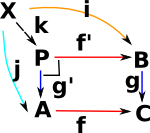P is the pullback of f along g or g along f (A,B,C fixed) .

Square must commute (in best possible way) so any other square, also containing (A,B,C) must uniquely map to it.P is the pushout of A,B,C

generalisation
• of limit
• of inverse
a kind of colimit
examples: set:

Σ<a,b>A×B| f(a)=g(b)

where:

k(x) maps to <j(x),i(x)>

called a fibred product.

A U B /equivalence relation

In concrete categories the Cartesian product is often the categorical product. The pullback is like the categorical product but with additional conditions. So in the following examples we have a Cartesian product with a subset of the rows and columns.

### Examples in Set

#### Set Example 1

 This diagram is both a pullback and a pushout.#### Set Example 2

How does the above example relate to the idea that the pullback is a subset of the cartesian product of two sets? We have this restriction on the Cartesian product:

Σ<a,b>A×B| f(a)=g(b)

 So, in this example, 'P' contains only the subset of the Cartesian product shown in red (that is ).#### Example in Number Expressions

This generates pairs of elements <a, b> : P with a in A, b in B, such that f(a) = g(b).

So the requirement for this square to commute generates in P all the possible pairs of numbers that are equal to each other.

So, in the example on the right, 4 is a 'representative' for all the things it is equal to such as 2+2 and 3+1. Sometimes the notation  is used for the set of all the things it is equal to (although this notation could be confused with list).### Examples in Graphs

• Pullbacks always exist.
• Pushouts do not necessarily exist.
 In this example only the red arrows go both ways round the square so only the red arrows exist in 'G'.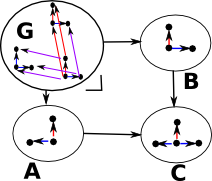### Examples in Groups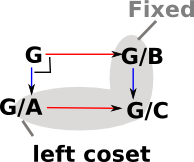see:

## Substitution as Pullback

Can we express substitution in category theory terms? See discussion on page here.

 Substitution of a term into a predicate is pullback, but substitution of a term into a term is composition. Type theory version Substitution of a term into a dependant type is pullback, but substitution of a term into a term is composition.

There is a discussion of this topic on this site.

So why the difference? I think in the predicate case we are going backwards.

 Typically a predicate could be an equation which may be true or false depending on the values of its variables. Or a function from a variable to bool (or some truth object).## Pullback as generalisation of Inverse

 Function/morphism from A to C is given. Subset B of C is given. Hence we have two morphisms to C so this looks like the given part of a pullback.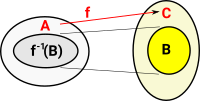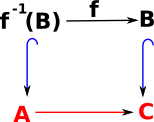To complete the square we add cone for this diagram and we call it f-1(B) to emphasise that it is the inverse of 'f'.

Reversing a function can generate interesting structure, for example, reversing addition on natural numbers leads to the integers and reversing multipication leads to fractional numbers.

 If we reverse a surjective mapping, where many elements in the domain map to a single element in the codomain, then reversing the function gives a fibre bundle.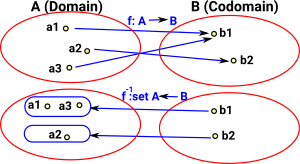If we reverse a injective mapping, where not all elements in the codomain are mapped to, then reversing the function gives a subobject structure.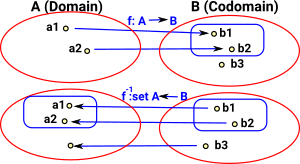## Relationship to Equaliser

 If the category A is the same as the category B then the pullback becomes an equaliser. See equalisers on this page.## Examples in Various Categories

Product
(pullback)generalisation   a kind of limit
set examplecartesian product

{a,b,c}*{x,y}=
{{a,x},{b,x},{c,x},{a,y},{b,y},{c,y}}

group   the product is given by the cartesian product with multiplication defined componentwise.
Grp (abelian)   direct sum
vector space   direct sum
poset   greatest lower bound
meet
base topological space
POS

greatest lower bounds (meets)

Rng
Top   the space whose underlying set is the cartesian product and which carries the product topology
Grf
category   objects: (a,b)
morphism: (a,b)->(a',b')

tensor products are not categorial products.

In the category of pointed spaces, fundamental in homotopy theory, the coproduct is the wedge sum (which amounts to joining a collection of spaces with base points at a common base point).

Catsters youtube videos - Terminal and initial objects

Catsters youtube videos - Products and coproducts

Catsters youtube videos - Pullbacks and pushouts

Catsters youtube videos - General limits and colimits

Where I can, I have put links to Amazon for books that are relevant to the subject, click on the appropriate country flag to get more details of the book or to buy it from them.The Princeton Companion to Mathematics - This is a big book that attempts to give a wide overview of the whole of mathematics, inevitably there are many things missing, but it gives a good insight into the history, concepts, branches, theorems and wider perspective of mathematics. It is well written and, if you are interested in maths, this is the type of book where you can open a page at random and find something interesting to read. To some extent it can be used as a reference book, although it doesn't have tables of formula for trig functions and so on, but where it is most useful is when you want to read about various topics to find out which topics are interesting and relevant to you.